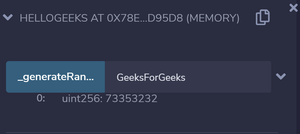GFG App
Open AppBrowser
Continue

# What is Hashing in Solidity?

A cryptographic hash function is an algorithm that takes an arbitrary amount of data as input and produces the enciphered text of fixed size. Even a slight change in the input gives a completely different output.

Solidity provides the following cryptographic functions:

Ethereum uses Keccak for hashing which is similar but not the same as SHA_256. For proof of work, it uses a custom scheme called ethash which is designed to be ASIC-resistant.

Example: In the below example, a smart contract is created to take a string as input and give an 8 digit hash as an output.

## Solidity

 `// pragma version ` `pragma solidity ^0.6.6;  ` ` `  `// Creating a contract ` `contract` `helloGeeks  ` `{ ` `    ``// We want hash to be of 8 digits  ` `    ``// hence we store 10^8 which is  ` `    ``// used to extract first 8 digits  ` `    ``// later by Modulus  ` `    ``uint hashDigits = 8; ` `     `  `    ``// Equivalent to 10^8 = 8 ` `    ``uint hashModulus = 10 ** hashDigits;  ` ` `  `    ``// Function to generate the hash value ` `    ``function _generateRandom(string memory _str)  ` `        ``public` `view returns (uint)  ` `    ``{ ` `        ``// "packing" the string into bytes and  ` `        ``// then applying the hash function.  ` `        ``// This is then typecasted into uint. ` `        ``uint random =  ` `             ``uint(keccak256(abi.encodePacked(_str))); ` `              `  `        ``// Returning the generated hash value  ` `        ``return` `random % hashModulus; ` `    ``} ` ` `  `}`

Input:

```GeeksForGeeks
```

Output:My Personal Notes arrow_drop_up